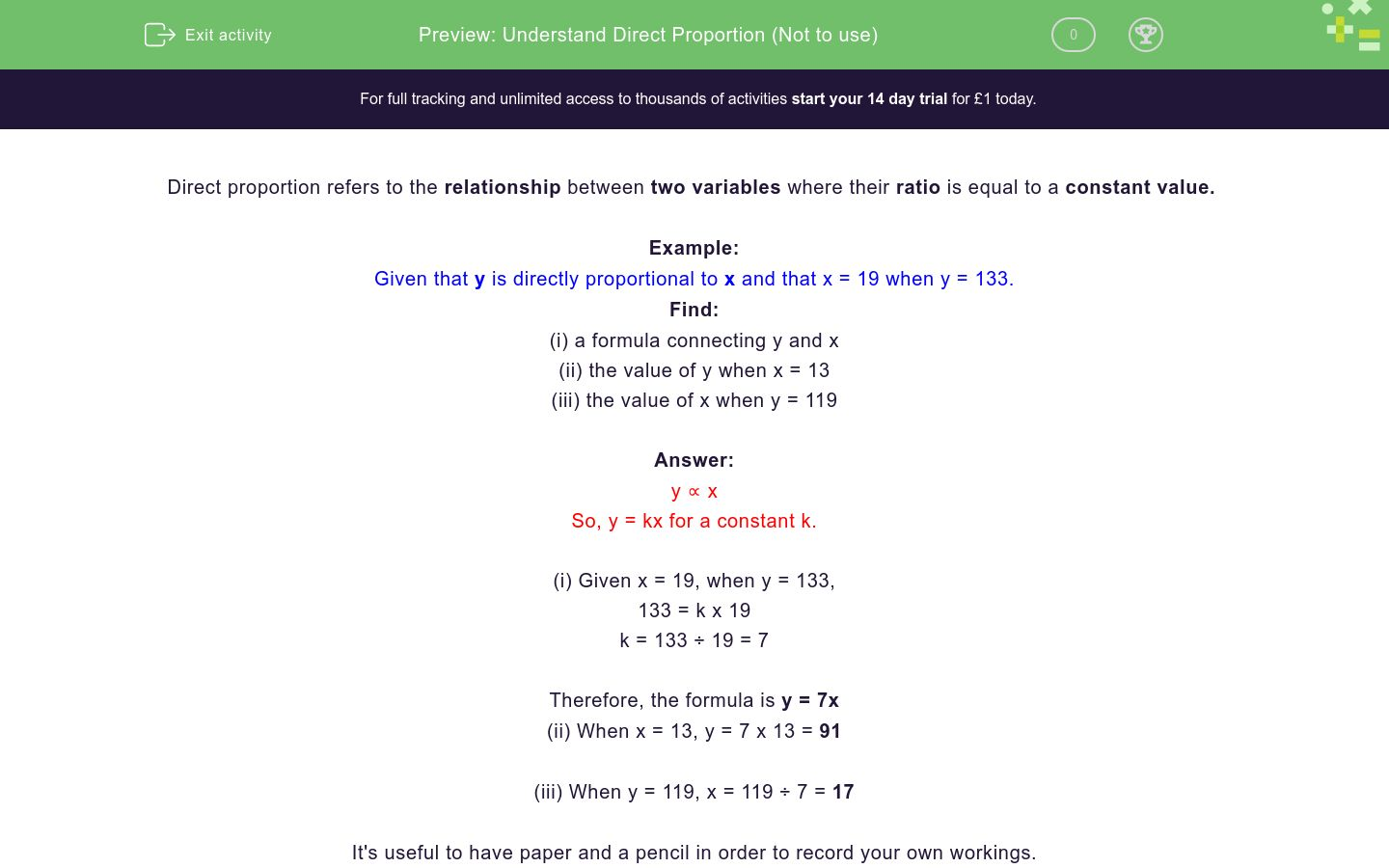# Direct Proportion

In this worksheet, students resolve problems related to direct proportion.Key stage:  KS 4

Curriculum topic:  Ratio, Proportion and Rates of Change

Curriculum subtopic:  Interpret Equations that Describe Direct and Inverse Proportion

Difficulty level:### QUESTION 1 of 10

This worksheet is about  direct proportion.

Example

Given that y is directly proportional to x and that x = 19 when y = 133.

Find:
(i) a formula connecting y and x
(ii) the value of y when x = 13
(iii) the value of x when y = 119

y ∝ x

so y = kx for a constant k.

(i) Given x = 19, when y = 133,

133 = k x 19

k = 133 ÷ 19 = 7

The formula is y = 7x

(ii) When x = 13, y = 7 x 13 = 91

(iii) When y = 119, x = 119 ÷ 7 = 17

Given that y is directly proportional to x and that x = 8 when y = 192.

Find a formula connecting y and x.

Given that y is directly proportional to x and that x = 8 when y = 192.

Find the value of y when x = 15.

Given that y is directly proportional to x and that x = 8 when y = 192.

Find the value of x when y = 600.

Given that y is directly proportional to x and that x = 7 when y = 105.

Find a formula connecting y and x.

Given that y is directly proportional to x and that x = 7 when y = 105.

Find the value of y when x = 9.

Given that y is directly proportional to x and that x = 7 when y = 105.

Find the value of x when y = 75.

Given that y is directly proportional to x and that x = 19 when y = 133.

Find a formula connecting y and x.

Given that y is directly proportional to x and that x = 19 when y = 133.

Find the value of y when x = 13.

Given that y is directly proportional to x and that x = 19 when y = 133.

Find the value of x when y = 119.

Given that y is directly proportional to x and that x = 12 when y = 192.

Find a formula connecting y and x.

• Question 1

Given that y is directly proportional to x and that x = 8 when y = 192.

Find a formula connecting y and x.

EDDIE SAYS
y ∝ x
y = kx
k = y/x = 192/8 = 24
y = 24x
• Question 2

Given that y is directly proportional to x and that x = 8 when y = 192.

Find the value of y when x = 15.

EDDIE SAYS
y = 24x
y = 24 × 15 = 360
• Question 3

Given that y is directly proportional to x and that x = 8 when y = 192.

Find the value of x when y = 600.

EDDIE SAYS
y = 24x
x= y/24 = 600/24
x = 25
• Question 4

Given that y is directly proportional to x and that x = 7 when y = 105.

Find a formula connecting y and x.

EDDIE SAYS
y ∝ x
y = kx
k = y/x = 105/7 = 15
y = 15x
• Question 5

Given that y is directly proportional to x and that x = 7 when y = 105.

Find the value of y when x = 9.

EDDIE SAYS
y = 15x
y = 15 × 9
y = 135
• Question 6

Given that y is directly proportional to x and that x = 7 when y = 105.

Find the value of x when y = 75.

EDDIE SAYS
y = 15x
x = y/15 = 75/15
x = 5
• Question 7

Given that y is directly proportional to x and that x = 19 when y = 133.

Find a formula connecting y and x.

EDDIE SAYS
y ∝ x
y = kx
k = y/x = 133/19 = 7
y = 7x
• Question 8

Given that y is directly proportional to x and that x = 19 when y = 133.

Find the value of y when x = 13.

EDDIE SAYS
y = 7x
y = 7 × 13
y = 91
• Question 9

Given that y is directly proportional to x and that x = 19 when y = 133.

Find the value of x when y = 119.

EDDIE SAYS
y = 7x
x = y/7 = 119/7
x = 17
• Question 10

Given that y is directly proportional to x and that x = 12 when y = 192.

Find a formula connecting y and x.

EDDIE SAYS
y ∝ x
y = kx
k = y/x = 192/12 = 16
y = 16x
Preview ---- OR ----

Sign up for a £1 trial so you can track and measure your child's progress on this activity.

### What is EdPlace?

We're your National Curriculum aligned online education content provider helping each child succeed in English, maths and science from year 1 to GCSE. With an EdPlace account you’ll be able to track and measure progress, helping each child achieve their best. We build confidence and attainment by personalising each child’s learning at a level that suits them.